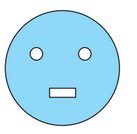Q

# From a circular card sheet of radius 14 cm, two circles of radius 3.5 cm and a rectangle of length 3 cm and breadth 1 cm are removed.

Q. 10.     From a circular card sheet of radius , two circles of radius and a rectangle of length and breadth are removed.(as shown in the adjoining figure). Find the area of the remaining sheet. (Take )Views

It is given that radius of circular card sheet is

Now, we know that

Area of circle (A) =

- (i)

Now,

Area of circle with radius 3.5 cm is

Area of two such circle is =                   -(ii)

Now, Area of rectangle =

-(iii)

Now, the remaining area is (i) - [(ii) + (iii)]

Therefore,  area of the remaining sheet  is

Exams
Articles
Questions